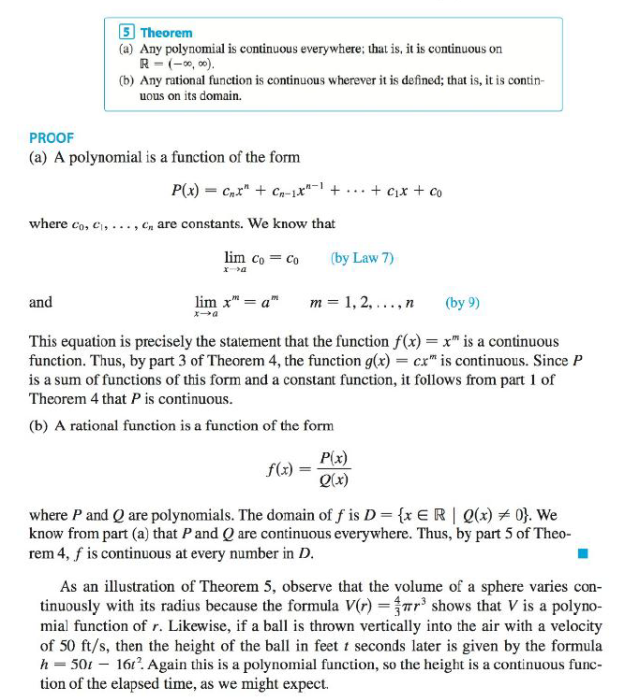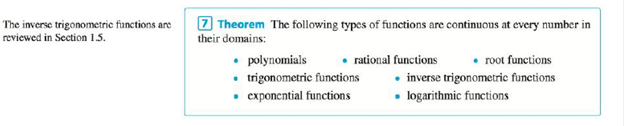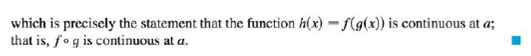Chapter 2.5, Problem 31E### Single Variable Calculus: Early Tr...

8th Edition
James Stewart
ISBN: 9781305270343

#### Solutions

Chapter
Section### Single Variable Calculus: Early Tr...

8th Edition
James Stewart
ISBN: 9781305270343
Textbook Problem

# Explain, using Theorems 4, 5, 7, and 9, why the function is continuous at every number in its domain. State the domain.Theorem 4Theorem 5Theorem 7Theorem 9M ( x ) = 1 + 1 x

To determine

To state: The domain and explain that the function is continuous at every number in its domain.

Explanation

Given:

The function M(x)=1+1x.

Theorems used:

5. (a) Any polynomial function is continuous everywhere; that is, it is continuous on =(,).

(b) Any rational function is continuous wherever it is defined; that is, it is continuous on its domain.

7. The functions such as “Polynomials, rational functions, root functions, trigonometric functions, inverse trigonometric functions, exponential functions and logarithmic functions” are continuous at every number in their domains.

9. If g is continuous at a and f is continuous at g(a), then the composite function fg given by (fg)(x)=f(g(x)) is continuous at a.

Calculation:

The domain is the set of all input values of the function for which the function is real and defined.

Consider the function M(x)=1+1x.

Simplify M(x) as follows,

M(x)=x+1x

The function M(x) is defined whenever x+1x must be greater than or equal to zero.

If x+1x0, then x+10 and x>0 or x+10 and x<0.

This implies that, x>0 or x1.

That is, if x+1x0 then x(,1](0,)

### Still sussing out bartleby?

Check out a sample textbook solution.

See a sample solution

#### The Solution to Your Study Problems

Bartleby provides explanations to thousands of textbook problems written by our experts, many with advanced degrees!

Get Started

#### Find more solutions based on key concepts